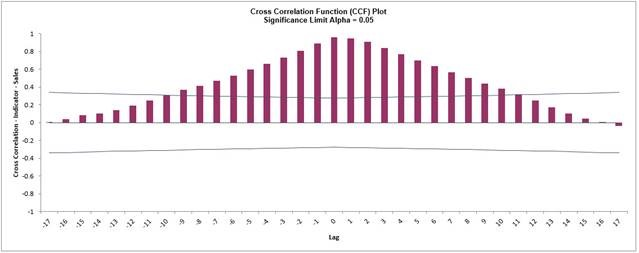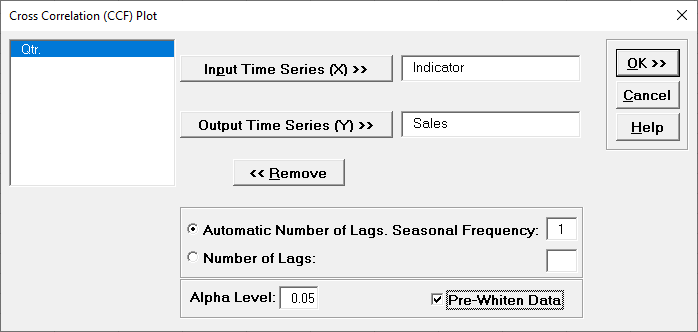Include Top

# Cross Correlation (CCF) Plots

Cross Correlation is similar to autocorrelation, but the correlations are computed on two related time series variables, typically a process input and output. A plot of the X data vs. the Y data at lag 𝑘 may show a positive or negative trend. If the slope is positive, the cross correlation is positive; if there is a negative slope, the cross correlation is negative. This helps to identify important lags (or leads) in the process and is useful for application when there are predictors in an ARIMA model.

If the X input is autocorrelated, the CCF is affected by its time series structure and any “in common” trends the X and Y series may have over time. Pre-whitening solves this problem by removing the autocorrelation and trends.

1. Open Sales with Indicator - Modified Series M.xlsx (Sheet 1 tab). This is modified Series M data from Box and Jenkins, with 50 quarters of corporate sales values along with a leading indicator. The data is treated as nonseasonal, as done in Box and Jenkins.
2. Click SigmaXL > Time Series Forecasting > Cross Correlation (CCF) Plot. Ensure that the entire data table is selected. If not, Use Entire Data Table. Click Next.
3. Click Indicator, click Input Time Series (X) >>. Select Sales, click Output Time Series (Y) >>. Use the default Automatic Number of Lags. Seasonal Frequency = 1 and Alpha Level = 0.05.4. Click OK. The CCF plot is produced.This CCF plot shows significant cross correlation from lag = -9 to +9, with a peak at 0, however the autocorrelation in X and Y data is masking the true nature of the cross correlation.

5. We will redo the CCF Plot with the Pre-Whiten Data option. Click Recall SigmaXL Dialog menu or press F3 to recall last dialog. Check Pre-Whiten Data.6. Click OK. The CCF plot is produced.Pre-Whitening the data has dramatically altered the CCF plot, allowing us to see the underlying cross correlation pattern. Lags 1 and 2 are significantly positive, and Lag 3 is just on the significance line.

Note that while X is called a leading indicator, i.e., X comes before Y in time, the positive lag means that the X variable is lagging the Y variable in terms of correlation structure. SigmaXL uses this convention as given in Box and Jenkins (2016, pp. 437-440).

This CCF plot will be useful later when we model the Sales data using ARIMA Forecast with Predictors.

# Web Demos

Our CTO and Co-Founder, John Noguera, regularly hosts free Web Demos featuring SigmaXL and DiscoverSim